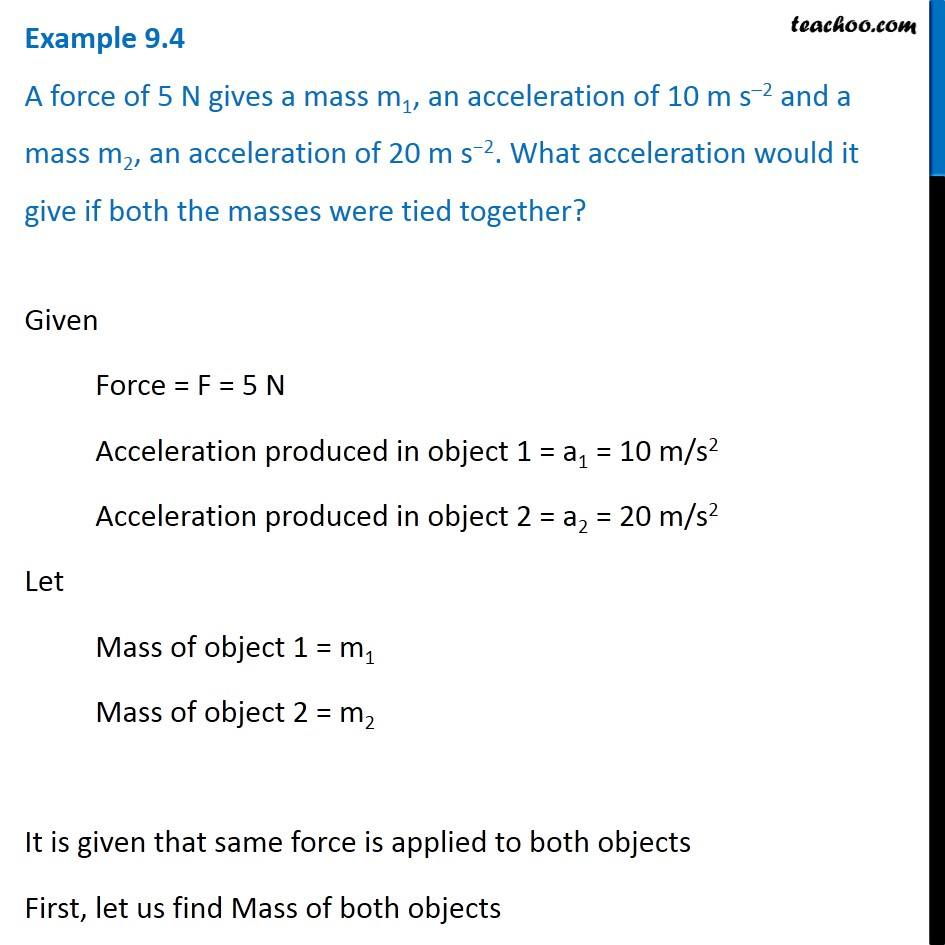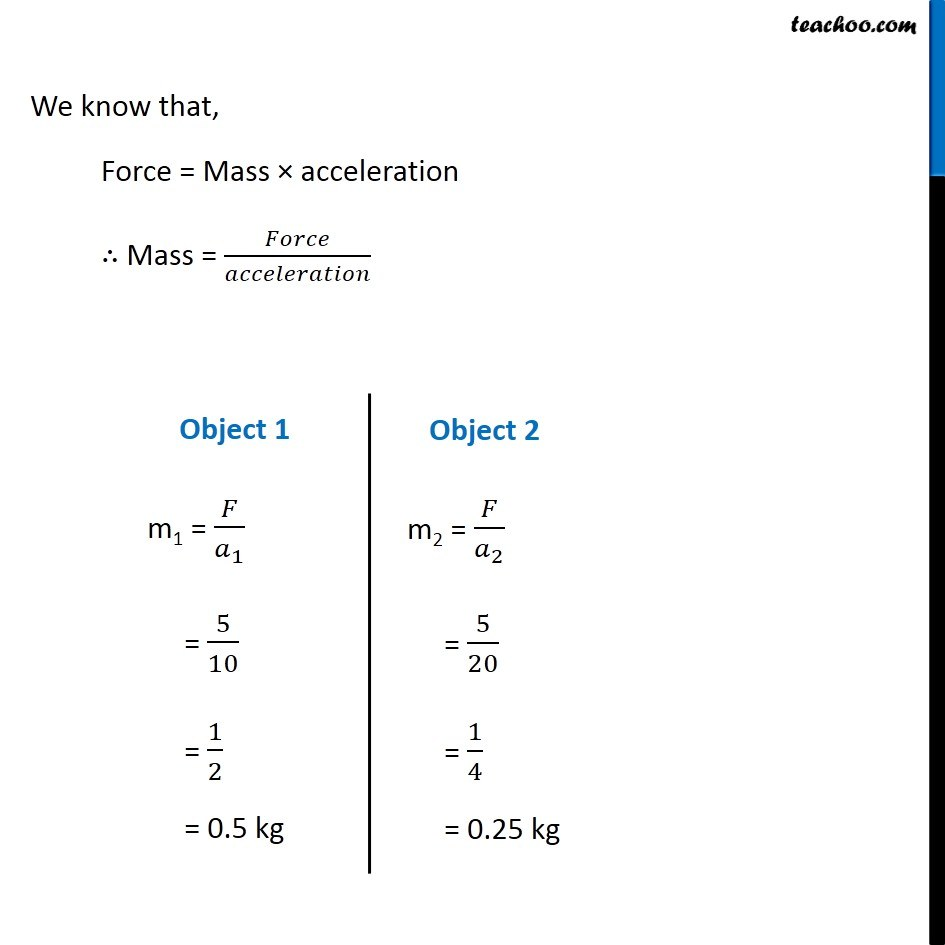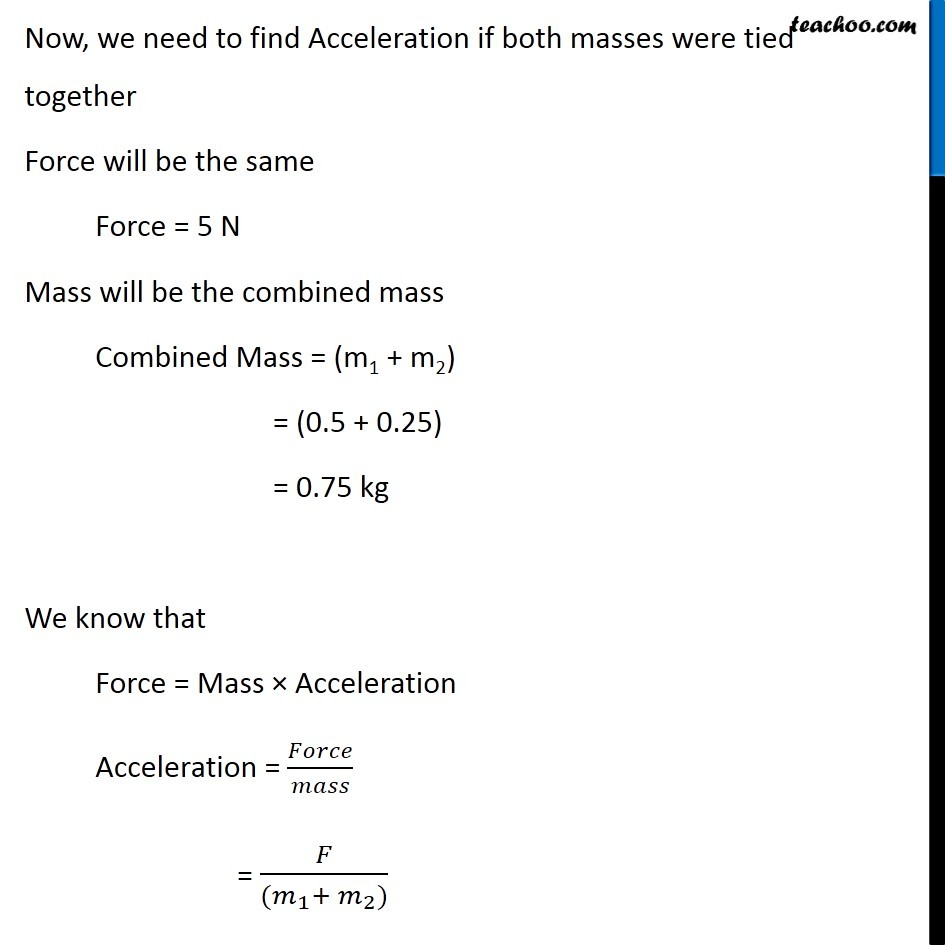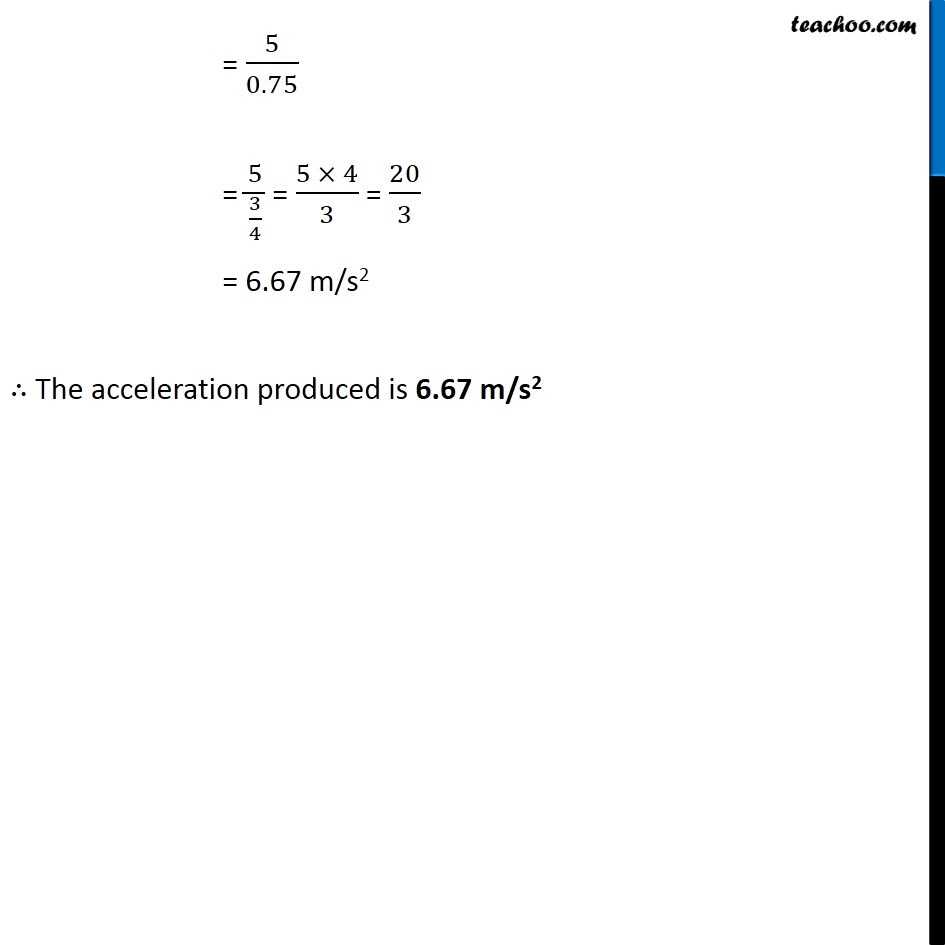Examples from NCERT Book

Class 9
Chapter 9 Class 9 - Force and Laws Of MotionLearn in your speed, with individual attention - Teachoo Maths 1-on-1 Class

### Transcript

Example 9.4 A force of 5 N gives a mass m1, an acceleration of 10 m s–2 and a mass m2, an acceleration of 20 m s−2. What acceleration would it give if both the masses were tied together? Given Force = F = 5 N Acceleration produced in object 1 = a1 = 10 m/s2 Acceleration produced in object 2 = a2 = 20 m/s2 Let Mass of object 1 = m1 Mass of object 2 = m2 It is given that same force is applied to both objects First, let us find Mass of both objects We know that, Force = Mass × acceleration ∴ Mass = 𝐹𝑜𝑟𝑐𝑒/𝑎𝑐𝑐𝑒𝑙𝑒𝑟𝑎𝑡𝑖𝑜𝑛 Object 1 m1 = 𝐹/𝑎_1 = 5/10 = 1/2 = 0.5 kg Object 2 m2 = 𝐹/𝑎_2 = 5/20 = 1/4 = 0.25 kg Now, we need to find Acceleration if both masses were tied together Force will be the same Force = 5 N Mass will be the combined mass Combined Mass = (m1 + m2) = (0.5 + 0.25) = 0.75 kg We know that Force = Mass × Acceleration Acceleration = 𝐹𝑜𝑟𝑐𝑒/𝑚𝑎𝑠𝑠 = 𝐹/((𝑚_1+ 𝑚_2)) = 5/0.75 = 5/(3/4) = (5 × 4)/3 = 20/3 = 6.67 m/s2 ∴ The acceleration produced is 6.67 m/s2